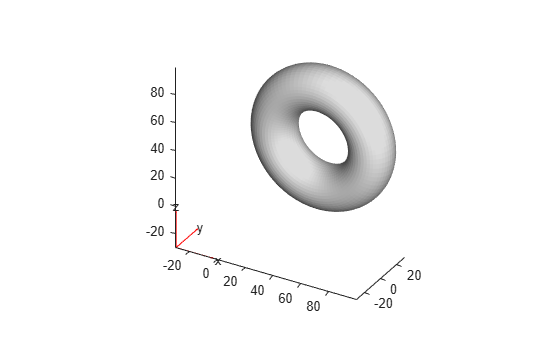# Assess Quality of Mesh Elements

Partial Differential Equation Toolbox™ uses the finite element method to solve PDE problems. This method discretizes a geometric domain into a collection of simple shapes that make up a mesh. The quality of the mesh is crucial for obtaining an accurate approximation of a solution.

Typically, PDE solvers work best with meshes made up of elements that have an equilateral shape. Such meshes are ideal. In reality, creating an ideal mesh for most 2-D and 3-D geometries is impossible because geometries have tiny or narrow regions and sharp angles. For such regions, a mesh generator creates meshes with some elements that are much smaller than the rest of mesh elements or have drastically different side lengths.

As mesh elements become distorted, numeric approximations of a solution typically become less accurate. Refining a mesh using smaller elements produces better shaped elements and, therefore, more accurate results. However, it also can be computationally expensive.

Checking if the mesh is of good quality before running an analysis is a good practice, especially for simulations that take a long time. The toolbox provides the `meshQuality` function for this task.

`meshQuality` evaluates the shape quality of mesh elements and returns numbers from 0 to 1 for each mesh element. The value 1 corresponds to the optimal shape of the element. By default, the `meshQuality `function combines several criteria when evaluating the shape quality. In addition to the default metric, you can use the `aspect-ratio` metric, which is based solely on the ratio of the minimum dimension of an element to its maximum dimension.

Create a PDE model.

`model = createpde;`

Include and plot the torus geometry.

```importGeometry(model,"Torus.stl"); pdegplot(model)```Generate a coarse mesh.

`mesh = generateMesh(model,Hmax=10);`

Evaluate the shape quality of all mesh elements.

`Q = meshQuality(mesh);`

Find the elements with quality values less than 0.5.

`elemIDs = find(Q < 0.5);`

Highlight these elements in blue on the mesh plot.

```figure pdemesh(mesh,FaceAlpha=0.5) hold on pdemesh(mesh.Nodes,mesh.Elements(:,elemIDs), ... FaceColor="blue",EdgeColor="blue")```Determine how much of the total mesh volume belongs to elements with quality values less than 0.5. Return the result as a percentage.

`mv03_percent = volume(mesh,elemIDs)/volume(mesh)*100`
```mv03_percent = 0.0892 ```

Evaluate the shape quality of the mesh elements by using the ratio of minimal to maximal dimension for each element.

`Q = meshQuality(mesh,"aspect-ratio");`

Find the elements with quality values less than 0.5.

`elemIDs = find(Q < 0.5);`

Highlight these elements in blue on the mesh plot.

```figure pdemesh(mesh,FaceAlpha=0.5) hold on pdemesh(mesh.Nodes,mesh.Elements(:,elemIDs), ... FaceColor="blue",EdgeColor="blue")```